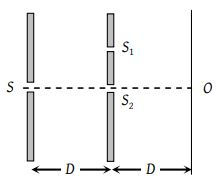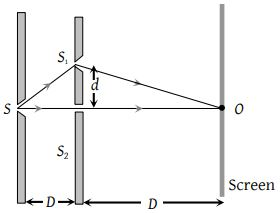Two ideal slits S1 and S2 are at a distance d apart, and illuminated by light of wavelength λ passing through an ideal source slit S placed on the line through S2 as shown. The distance between the planes of slits and the source slit is D. A screen is held at a distance D from the plane of the slits. The minimum value of d for which there is darkness at O is(1) $\sqrt{\frac{3\lambda D}{2}}$

(2) $\sqrt{\lambda D}$

(3) $\sqrt{\frac{\lambda D}{2}}$

(4) $\sqrt{3\lambda D}$

(3) Path difference between the waves reaching at P, $\Delta ={\Delta }_{1}+{\Delta }_{2}$

where Δ1 = Initial path differenceΔ2 = Path difference between the waves after emerging from slits.

${\Delta }_{1}=S\text{\hspace{0.17em}}{S}_{1}-S\text{\hspace{0.17em}}{S}_{2}=\sqrt{{D}^{2}+{d}^{2}}-D$

and ${\Delta }_{2}={S}_{1}O-{S}_{2}O=\sqrt{{D}^{2}+{d}^{2}}-D$

$\therefore \text{\hspace{0.17em}\hspace{0.17em}\hspace{0.17em}}\Delta =2\left\{{\left({D}^{2}+{d}^{2}\right)}^{\frac{1}{2}}-D\right\}=2\left\{\left({D}^{2}+\frac{{d}^{2}}{2D}\right)-D\right\}$

$=\frac{{d}^{2}}{D}$(From Binomial expansion)

For obtaining dark at O, Δ must be equals to $\left(2n-1\right)\frac{\lambda }{2}$ i.e. $\frac{{d}^{2}}{D}=\left(2n-1\right)\frac{\lambda }{2}⇒d\sqrt{\frac{\left(2n-1\right)\lambda \text{\hspace{0.17em}}D}{2}}$

For minimum distance n = 1 so $d=\sqrt{\frac{\lambda \text{\hspace{0.17em}}D}{2}}$

Difficulty Level:

• 19%
• 26%
• 40%
• 17%